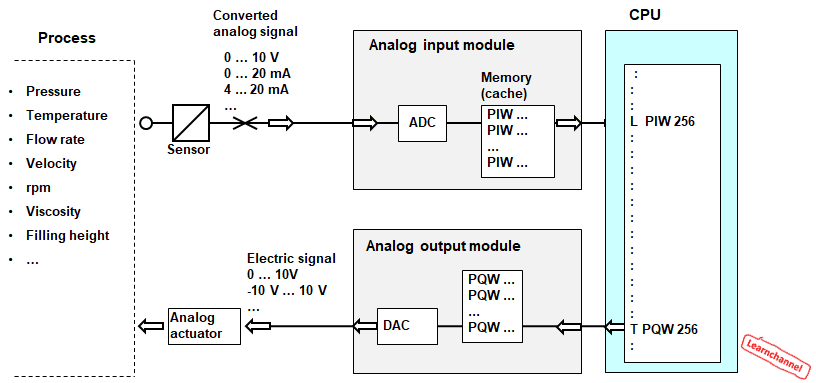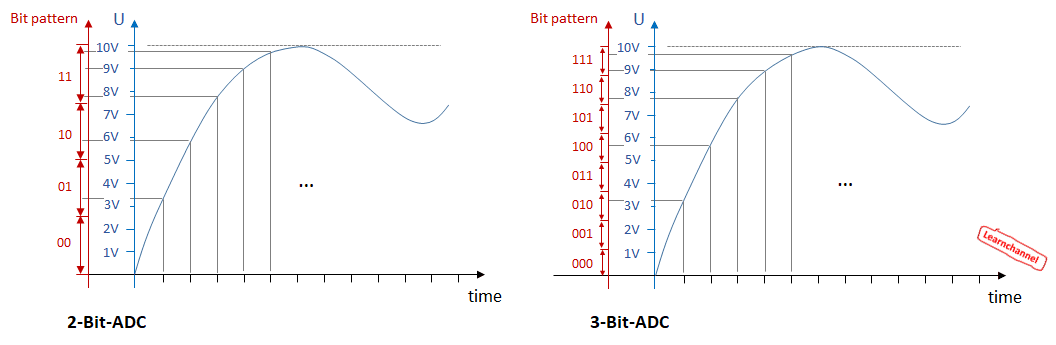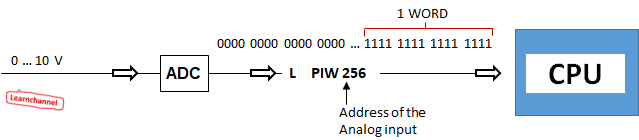# Analog value processing

## Analog value processing

In a production process there are a large number of physical variables (pressure, temperature, speed, rotational speed, viscosity, etc.). These so-called analog signals must be processed by the PLC. The following figure demonstrates analog signal processing in a PLC:PLC - Analog value processing - Use of Analog Modules

Terms:

 Sensor Sensor elements are also called ‘converters’ because they convert a physical quantity (in most cases a mechanical quantity) into an electrical signal, such as current, voltage or electrical resistance. ADC Before the analog values ​​can be processed from the CPU, they must be converted in a digital form. This is done by the ADC (Analog-Digital Converter) within the analog input module. Input / output cache The result of the conversion is stored in a cache and stays there until it is overwritten by a new value. The converted analog value can be read with the instruction load "L PIW ...” Analog output The transfer instruction "T PQW ..." is used to write the analog value calculated by the user program in the analog output, where a DAC (Analog-Digital Converter) module converts the values for standard analog signals.

### How a ADC converter (Analog-Digital-Converter) works:

Analog signals need to be converted to digital information before being processed by the PLC. The module, which performs the analog to digital conversion is named as ADC (Analog-Digital-Converter).

This means that, for example, the voltage of 10V is stored in a series of bits as binary information. The more binary digits are available, the better the resolution.Working principle of an Analog-Digital-Converter

So if e.g. for the voltage range of 0 ... 10 V only 2 bits are available, a voltage range can be divided into four separate ranges: 0 ... 2.5 / 2.5 ... 5/5 ... 7.5 / 7.5 ... 10V. The resolution can be determined with the following equation:

Resolution = Range of measurement        (n = Number off bits)
2n

If a distinction is still to be made between negative and positive voltage, one bit must be reserved for the sign of the voltage. The equation changes to:

Resolution = Range of measurement        (n = Number off bits)
2n - 1

A commonly used A/D converter in the industry has between 8 and 11 bits. With 8 bits you get 256 steps, by an 11-bit ADC already 2048 steps.

The analog values ​​are read in by the input module and send to the CPU as an information in format "WORD", that means 16 Bits. The instructions to get access to this data are the following:

L PIW    xxx       //      load process-input WORD into the CPU (Load Process Input Word)
T PQW  xxx        //     transfer process-output WORD to the Analog output module (Transfer Process Input Word)PLC - Analog Signal Processing